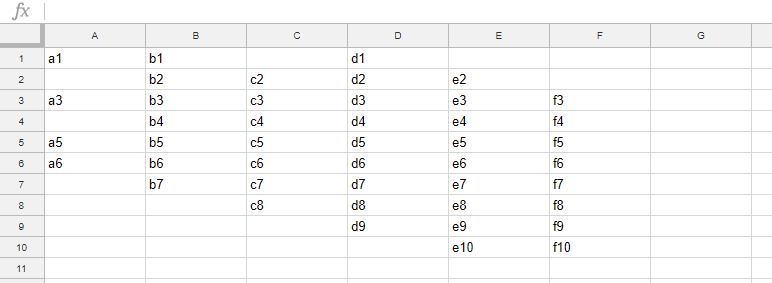# Retrieve Last of Specific Row and Column

Gists

This is a sample script for retrieving the last coordinate of the specific row and column. When the methods of getLastRow() and getLastColumn() of Class Range for Spreadsheet are used, the last coordinates of the vertical and horizontal data range can be retrieved. When users want to retrieve the last coordinates of each row and column, there are no methods. So I created this script. I think that there are several scripts for this situation. So please think of this as one of them. If this was useful for you, I’m glad.

As a sample situation, it supposes the following sheet and the container-bound script. If the formulas are included in the row and column you want to retrieve the last, this script can also check them.## Script

function getLast(range) {
var getResult = function(range) {
if (!((range.getNumRows() > 1 && range.getNumColumns() == 1) || (range.getNumRows() == 1 && range.getNumColumns() > 1))) {
throw new Error("Please input one row or one column.");
}
var v = Array.prototype.concat.apply([], range.getValues());
var f = Array.prototype.concat.apply([], range.getFormulas());
var i;
for (i = v.length - 1; i >= 0; i--) {
if (v[i] != "" || f[i] != "") break;
}
return i + 1;
};
if (Array.isArray(range)) {
return range.map(function(e) {
return getResult(e);
});
} else {
try {
range.getA1Notation();
} catch (e) {
throw new Error("Inputted value is not a range.");
}
return getResult(range);
}
}

function main() {

var range1 = sheet.getRange("A:A");
var r1 = getLast(range1); // Retrieve last row of column 1.
Logger.log(r1); // 6

var range2 = sheet.getRange("1:1");
var r2 = getLast(range2); // Retrieve last column of row 1.
Logger.log(r2); // 4

var range3 = sheet.getRangeList(["A:A", "1:1"]).getRanges();
var r3 = getLast(range3); // Retrieve both last row of column 1 and last column of row 1.
Logger.log(r3); // [6.0, 4.0]
}


Please give “range” to getLast(). “range” and “rangelist” can be used for this script as the sample script.